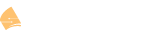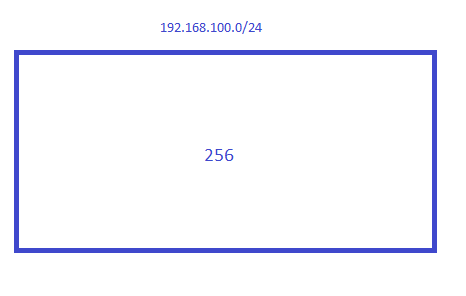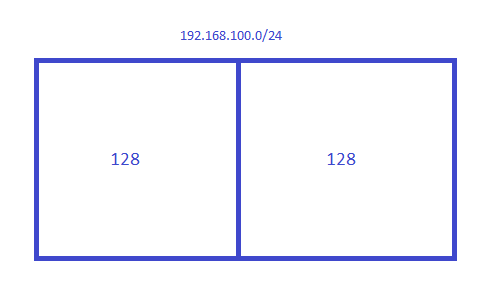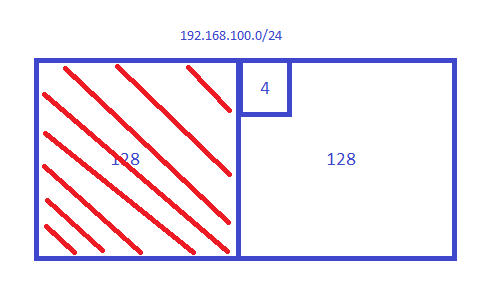# Subnetting in Decimal (Fast Way)

(Rene Molenaar) #6

Thanks for letting me know, just fixed them!

(ARNOLD T) #7

great information, i have been in a class room setting for a bit and never had it explained this way

(PALANIAPPAN M) #8

Hi Rene
When creating this blocks, do they have to be of equal size?

For example, you have an address of 10.10.10.0/23, and I am working to create a network/mask for the below requirements

I. 24 hosts
II. 111 hosts
III. 47 hosts
IV. 200 hosts

I came up with this , can you let me know if this is correct

I. 10.10.10.0/27
II. 10.10.10.128/25
III. 10.10.10.64/26
IV. 10.10.11.0/24

If I did this way, is this correct?

I. 10.10.10.192/27
II. 10.10.11.64/25
III. 10.10.11.0/26
IV. 10.10.10.0/24

Thanks
Palani

(Andrew P) #9

Palani,
Short answer: Everything you have is correct. For any given subnet mask, the block size is always the same.

People do subnetting many different ways. I haven’t heard of others doing it my way, but it works for me. I thought I might share with you how I can do subnetting very fast in my head–no calculators or even pencil and paper required.

I use the formula 2^X >= Y, which reads as 2 to the Xth power is greater than or equal to Y. Y is the number of hosts (or subnets) you are trying to figure out, and X is the variable you are trying to solve for. Let’s walk through one of your examples: 47 hosts. In this case we know that Y = 47. So now we have to figure out what power of 2 is as close to 47 as possible, but cannot be less. Two to the fifth power is 32, so that’s too small. How about 6? Two to the sixth power is 64–so that’s our answer: X = 6.

So what does X do for you? A lot! Once you solve for X, you can easily figure out two things:

1. The network block size (the range of each subnet): This is simply 2^X, which in our case is 64. This means that your subnet ranges would be…
0 - 63
64 - 127
128 - 191
192 - 255
Notice how on the left column above, I just increase the number by the block size? Easy!

2. The subnet mask. You will have to know which octet you are trying to figure out (in this case it is the last octet). To figure out the mask, simply subtract 2^X from 256–so in this case it would be: 256 - (2^6) = 256 - 64 = 192. Now you know the subnet mask will be 255.255.255.192. Easy!

(James D) #10

Both Rene and Andrew are amazing. I have been stuck on this for a couple of days and all I can say is THANK YOU!! The 2^x>=Y method and the 256 - block size just about cleared all my questions. Being a full-time worker, college student, and Dad is a lot on my plate and sometimes my brain is too tired to grasp things from the textbooks and lectures. I looked at this site today and viola, I am confident now.

Jim D.

(Jeremy C) #11

It would help if you included the subnet mask or prefix in your examples.

(Rene Molenaar) #12

Hi Jeremy,

In this example we do show the subnet mask for each “block”?Rene

(Gerson F) #13

Hi gyus,

I’ve done the exercises and I am most certain that they are correct, but could you leave us a link or an appendix with the solutions for confirmation purposes?

Regards,
Gerson

(Rene Molenaar) #14

Hi Gerson,

A subnet calculator might be even more useful. You can use this to verify anything you calculated.

For example:

http://www.subnet-calculator.com/

Rene

(Rahul A) #15

Hi Andrew,

If i require just 2 hosts for P2P, i am having trouble using your formula. Since 2^X >= Y, where Y is 2, which makes X = 1. Now with X=1, make 2^1=2. The Subnet ranges would be:
0-1
2-3
and so on.
Which I believe is not correct, since for 192.168.1.0/30 subnet, the host would be 192.168.0.1 - 192.168.0.2. Please clarify?

(Andrew P) #16

Use the formula (2^X) - 2 >= Y

(Maros Z) #17

Guys, I need help. Every time I try getting into different topics, be it NAT, Routing Policy, etc - I always get back to Subnetting. I finally realized there’s no way to progress without mastering this subject. I google everywhere, but I need your help and hopefully I’ll finally figure this out. Please, show me how would you answer this question;

Write a standard ACL that will cover the host range from 192.168.100.128 through 192.168.100.131.

Let’s not worry about the ACL part, but how would you go about writing an IP address with a subnet (wildcard) mask that would cover the need for 3 usable hosts?

You’d really help me out a lot.

Thank you in advance.

(Rene Molenaar) #18

Hi Maros,

Once you understand the fundamentals of subnetting, a quick way to solve a question like this is to visualize it and think in “blocks”:

And take a look at VLSM:

We want an access-list that matches four addresses, it starts with 192.168.100.128:

• 192.168.100.128
• 192.168.100.129
• 192.168.100.130
• 192.168.100.131

The first thing we need to figure out is what these addresses are. Is it an exact subnet? In this case, it might be easy to see but if someone asks you to block “192.168.100.44/29”, you’ll need to figure this out. A quick way to find out is to visualize the 192.168.100.0/24 subnet as a “block” of 256 addresses:If I cut it in half, I get two blocks:You get two blocks of 128 so there are two subnets:

• Subnet 1: 192.168.100.0 - 192.168.100.127
• Subnet 2: 192.168.100.128 - 192.168.100.255

This quickly tells me that 192.168.100.128 is a network address and since you specify four addresses, (a block of four), we know it’s an exact subnet that you try to match.

The range you want to match is not in the first block so we can forget about it. It is on the second block though:So what’s the subnet mask for your subnet? A quick way to figure that out is to take the number 256 minus the block size:

256 - 4 = 252

We are working in the fourth octet so your subnet mask is 255.255.255.252

Now since we need a wildcard instead of a subnet mask, we have to convert it. In binary, the subnet mask looks like this:

11111111 11111111 11111111 11111100

A wildcard is an “inverted” subnet mask so we just flip the bits:

00000000 00000000 00000000 00000011

Which gives us a wildcard of 0.0.0.3

A quicker method is just to take the block size minus 1. Our subnet is a block of 4, so the wildcard becomes 3.

• If you have a subnet that has 8 addresses, the wildcard is 7.
• If you have a subnet that has 16 addresses, the wildcard is 15.
• Etc

These “tricks” make it easier to quickly answer questions like this but make sure you understand the fundamentals, otherwise, you have no idea why we use these tricks. If not, you might want to go over the binary examples first:

Hope this helps!

Rene

1 Like
(Charles A) #19

Hi Rene,

Thanks for the great work. You are really making the topics easy to understand. One observation I want you to clarify is what you said about every bit borrowed doubles you network. It is true for the 1st and the 2nd bit but does not appear to be true for 3 bits and up. Can you confirm?

(Rene Molenaar) #20

Hello Charles,

Glad to hear you like it!

This logic applies to all bits. Let’s look at an example for subnet 192.168.1.0/24. We need to look at the fourth octet where the host bits are. In binary it looks like this:

00000000

Right now we have 8 host bits and 1 subnet. Let’s borrow one host bit so we can create more subnets.

10000000

Now we have 2 subnets and 7 host bits. Let’s borrow one more:

11000000

Now we have 4 subnets and 6 host bits. Let’s borrow one more:

11100000

Now we have 8 subnets and 5 host bits. Let’s borrow one more:

11110000

Now we have 16 subnets and 5 host bits. You can continue like this.

Does this make sense?

Rene

(Jose S) #21

This is DOPE! Rene is a real beast!

1 Like
(Ajeet K) #22

Hi Rene,

I see that you have mentioned that 192.168.1.0 is the first subnet and 192.168.1.128 is the second subnet. I am confused as to why we didn’t we use 192.168.0.0 as first subnet. Why is the ZERO subnet missing?? Please help.

(Lazaros Agapides) #23

Hello Ajeet

When doing subnetting, the first thing you define is the full range of addresses that you begin with. This is typically what the ISP will give you or what you have to work with within your network. From this range of addresses, you begin subnetting into smaller subnets.

In Rene’s example, the original network range that you are given is the 192.168.1.0 subnet with a mask of 255.255.255.0. This is provided by definition. You are then asked to subnet this range. In the lesson, the you are initially asked to use a subnet mask of 255.255.255.128. So by definition again, the very first subnet will be 192.168.1.0 because that is what you have been given and the second will be 192.168.1.128.

Now if you were given the initial range of 192.168.2.0/23 and asked for example to use a subnet mask of 255.255.255.192, then the first subnet would be 192.168.2.0, again because that’s what you are given to start with. The next would be 192.168.2.64, then 192.168.2.128 etc…

I hope this has been helpful!

Laz

(Ajeet K) #24

Thank you for replying Lazaros.

In your second paragraph, you have mentioned that if we were to subnet 192.168.1.0/24 to subnet mask /25, then the first subnet should be 192.168.1.0. Why can’t we take the first subnet as 192.168.1.128 - This can be the network address and the broadcast address can be 192.168.1.255.
Also, I think in the first line of 3rd paragraph, it should be 192.168.2.0/26. Please correct me if I am wrong.
Adding to it, I would also like to know if VLSM is enabled on all the routers by default or we have to enable it. Because, I guess the router will assume fixed size subnets if we don’t enable VLSM.

Thanks

(Lazaros Agapides) #25

Hello Ajeet

If you are originally given a range of 192.168.1.0/24, and you begin dividing it into smaller subnets, the first subnet is always the lowest. So if we begin dividing this into /28 subnets for example, the first subnet will be 192.168.1.0/28. The second will be 192.168.1.16/28, then 192.168.1.32 and so on. Of course, each subnet has its network address, first, last and broadcast addresses as well…

Actually, I meant /23. 192.168.2.0/23 gives us a range of addresses from 192.168.2.0 to 192.168.3.255.
If we subdivide this into smaller subnets using the 255.255.255.192, then we get the following subnets:

192.168.2.0/26
192.168.2.64/26
192.168.2.128/26
192.168.2.192/26
192.168.3.0/26
192.168.3.64/26
192.168.3.128/26
192.168.3.192/26

The example I used was to show that you start with an original range, and depending on the sizes of the subnets you want to make, you can divide that into smaller pieces.

I hope this has been helpful!

Laz